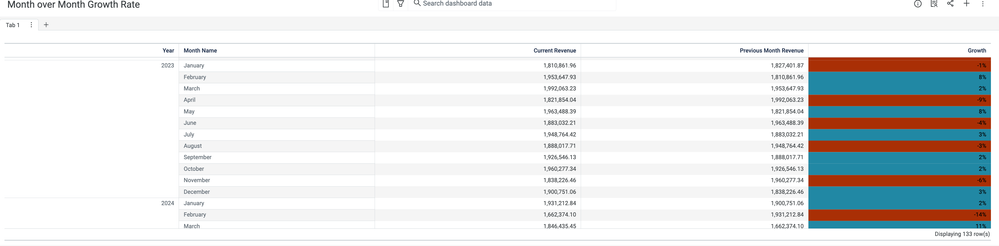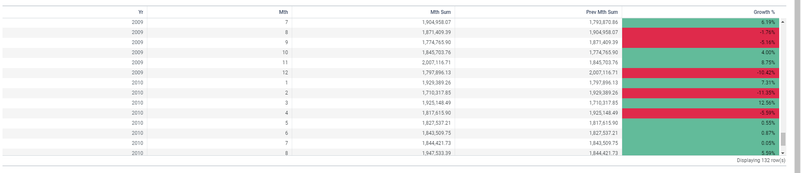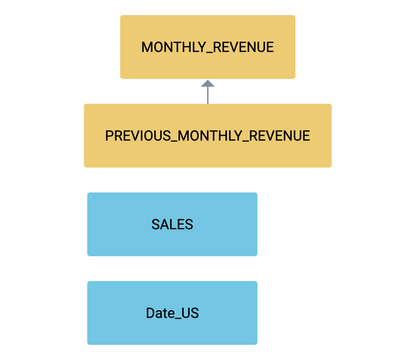cancel
Showing results for
Did you mean:

## Challenge #3 Month over Month Growth RateEmployee

As a Sales Analyst, I need your help to know how my company's revenue grows throughout a period using the month-over-month growth formula :

(Month growth rate = (This month - Last month) / Last month)

I am looking for this insight:You can use the attached Sales data and Date lookup data.

I know it is a little bit tricky, But  When you try it you will find it is fun 🙂

7 REPLIES 7Partner

Hi Rasha,
My solution involved created a materialized view using the Spark SQL feature which created a table that showed , Year, Month, Mth Sum, and Previous Month Sum. I then loaded that Schema in and created a formula inside the insight to show the growth percentage. Im interested to see if you had the same solution. Ive attached my graphic below.Community Manager

Great stuff @TCoomes !Employee

Not the most elegant, but I got the end result! Here is my solution:

1. Create analyzer table named MONTHLY_REVENUE with 3 columns: Year, Month, Revenue
2. Create analyzer table named PREVIOUS_MONTHLY_REVENUE with 3 columns: Year, Month, Previous Month Revenue
3. Join Tables on Year and Month
4. Create visualization with the Year, Month, Revenue, Previous Month Revenue, and Growth Rate using the formula provided.

In step 2, you can represent the previous month revenue by adjusting the month by 1 year. After doing so, the sum of revenue will represent the previous month.

Excited to see some other solutions!Employee

``````To calculate the month-over-month growth rate in Incorta, you will need to perform the following steps:

1. Join the `sales` and `months` tables on the `TIME_ID` column in the `sales` table and the `Date` column in the `months` table.
2. Create a calculated column in the joined table to calculate the revenue for each month by multiplying the `QUANTITY_SOLD` by the `AMOUNT_SOLD`.
3. Create two calculated columns in the joined table to calculate the revenue for each month and the previous month. To do this, you can use the following steps:
a. Use the `Window` function to create a sliding window over the rows in the joined table, ordered by the `Date` column in the `months` table.
b. Use the `LAG` function to retrieve the revenue value for the previous month for each row in the joined table.
c. Use an `IF` statement to handle the case where there is no previous month for the first row in the joined table. In this case, you can set the revenue for the previous month to 0.
4. Create a calculated column in the joined table to calculate the month-over-month growth rate using the following formula:
`Month growth rate = (This month revenue - Last month revenue) / Last month revenue`
5. Group the data by the `Month_Name` column to see the revenue for each month.
6. Create a visualization such as a line chart or bar chart to display the month-over-month growth rate.

This is a more detailed outline of the steps needed to calculate the month-over-month growth rate in Incorta, including the exact formula that will be used. The specific details of the calculation will depend on the data and the requirements of your project.``````

Then asked him for more details on Step 3, and here it is:

``````To calculate the revenue for each month and the previous month in Incorta, you can use the following formula in a calculated column:

```
CASE
WHEN ROW_NUMBER() OVER (ORDER BY "Date") = 1 THEN 0
ELSE LAG("Revenue", 1, 0) OVER (ORDER BY "Date")
END
```

In this formula, the `ROW_NUMBER()` function is used to determine the row number for each row in the joined table, ordered by the `Date` column in the `months` table. The `LAG` function is used to retrieve the revenue value for the previous month for each row in the joined table. The `CASE` statement is used to handle the case where there is no previous month for the first row in the joined table. In this case, the revenue for the previous month is set to 0.

This formula can be used to create two calculated columns in the joined table, one for the revenue for each month and one for the revenue for the previous month.``````